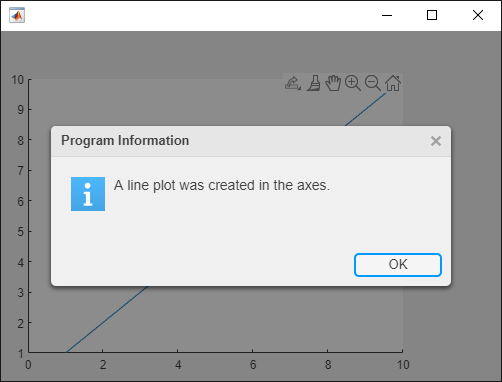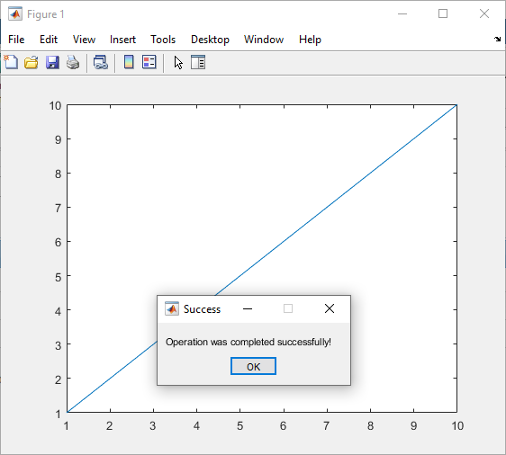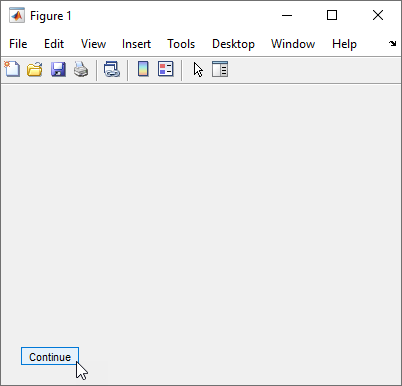# uiwait

## 语法

``uiwait``
``uiwait(f)``
``uiwait(f,timeout)``

## 说明

``uiwait` 阻止程序执行，直至调用了 `uiresume` 函数或删除了当前图窗 (`gcf`)。`uiwait` 函数阻止 MATLAB® 和 Simulink® 程序执行。`uiwait` 还阻止 Simulink 模型的执行。`

``uiwait(f)` 阻止程序执行，直至调用了 `uiresume` 函数或删除了图窗 `f`。该图窗可以是使用 `figure` 或 `uifigure` 函数创建的。使用 `uiwait` 函数和模态对话框可阻止程序执行并限制用户交互仅在对话框中进行，直到用户对它作出响应。`

``uiwait(f,timeout)` 阻止程序执行，直至调用了 `uiresume`、删除了图窗或经过了 `timeout` 秒。`

## 示例

```fig = uifigure; fig.Position = [500 500 500 350]; ax = uiaxes(fig); plot(ax,1:10) uialert(fig,'A line plot was created in the axes.', ... 'Program Information','Icon','info','CloseFcn','uiresume(fig)') uiwait(fig) disp('Program execution resumed')``````f = figure; plot(1:10) msgfig = msgbox('Operation was completed successfully!','Success','modal'); uiwait(msgfig) disp('Program execution resumed.');``````f = figure('Position',[500 500 400 300]); c = uicontrol('String','Continue','Callback','uiresume(f)'); uiwait(f) disp('Program execution has resumed');``````fig = uifigure; fig.Position = [500 500 500 350]; ax = uiaxes(fig); plot(ax,1:10);```

```uiwait(fig,5) try close(fig) catch end```